## 前言

• 基于向量的长度和夹角求叉乘
• 基于向量的坐标点求叉乘

### 1-基本公式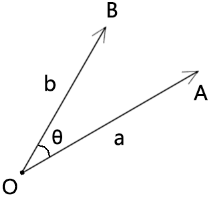• 向量a的长度是|a|
• 向量b的长度是|b|
• 向量a和向量b的夹角是θ

``````a^b=|a|*|b|*sinθ
``````

### 2-向量在轴上的正射投影

``````a^b=|a|*|b|*sinθ
``````

``````sinθ=y/r
y=r*sinθ
``````

• |b|是不是可以作为斜边？
• |b|*cosθ 是不是一条对边？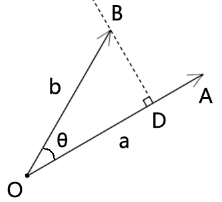``````BD=|b|*sinθ
``````

• 正射投影的值是个实数，可正可负。
• 两个向量的夹角的值域是[-180°,180°]
• 两个向量的夹角和叉乘的关系：
• 当θ=90° 时，b⊥a ，正射投影=|b|，叉乘=|a|*|b|
• 当θ=-90° 时，b⊥a ，正射投影=-|b|，叉乘=-|a|*|b|
• 当θ=0°或180° 时，正射投影=0，叉乘=0
• 当θ∈(0°,180°) 时，正射投影>0，叉乘>0
• 当θ∈(-180°,0°) 时，正射投影<0，叉乘<0
• a^b≤|a|*|b|，因为叉乘可正、可负、可零，|a|*|b|≥0

### 3-叉乘的运算律

#### 3-1-交换律

``````a^b=-b^a
``````

``````a^b=|a|*|b|*sin<a,b>
b^a=|b|*|a|*sin<b,a>
``````

``````a^b=-b^a
``````

``````a+b=b+a
A*b=b*a

a-b=-(b-a)
a^b=-b^a
``````

#### 3-2-分配率

``````(a+b)^c=a^c+b^c
``````

#### 3-3-其它公式

``````(a+b)²=a²+2ab+b²
(a+b)*(a-b)=a²-b²
```
```

### 1-基本公式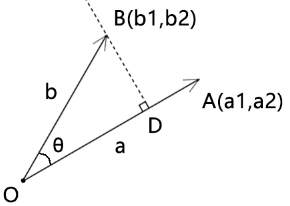• 向量a和向量b所在的坐标系为二维直角坐标系
• 向量a的坐标点位是(a1,a2)
• 向量b的坐标点位是(b1,b2)

``````a^b=a1*b2-a2*b1
``````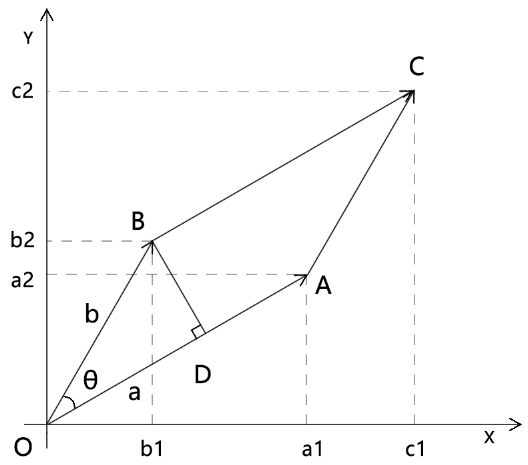• 向量OA=a=(a1,a2)
• 向量OB=b=(b1,b2)
• ∠<a,b>=θ

``````a^b=a1*b2-a2*b1
``````

``````S▱OACB=|a|*|b|*sinθ
``````

S▱OACB等于包含了▱OACB的矩形OC1CC1的面积，减去除▱OACB 之外的部分。

``````S▱OACB=(a1+b1)*(b2+a2)-b1*a2-a1*a2/2-b1*b2/2-b1*a2-b2*b1/2-a2*a1/2
S▱OACB=a1*b2+b1*b2+a1*a2+b1*a2-b1*a2-a1*a2/2-b1*b2/2-b1*a2-b2*b1/2-a2*a1/2
S▱OACB=a1*b2+b1*b2+a1*a2+b1*a2-2*b1*a2-a1*a2-b1*b2
S▱OACB=a1*b2-b1*a2
``````

``````a^b=a1*b2-b1*a2

``````

### 实例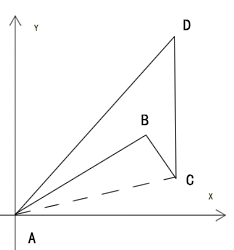``````S△ACD-S△ABC
``````

``````S△ABC=AB^AC/2
``````

``````-90°<∠BAC<0
90°>∠CAB>0
``````

``````sin∠BAC<0
sin∠CAB>0
``````

``````AB^AC=|AB|*|AC|*∠BAC
``````

``````AB^AC<0
``````

``````(AB^AC+AC^AD)/2

``````

`多边形面积 = (a^b + b^c + ... + f^g)/2`

## 发表评论

•• 匿名